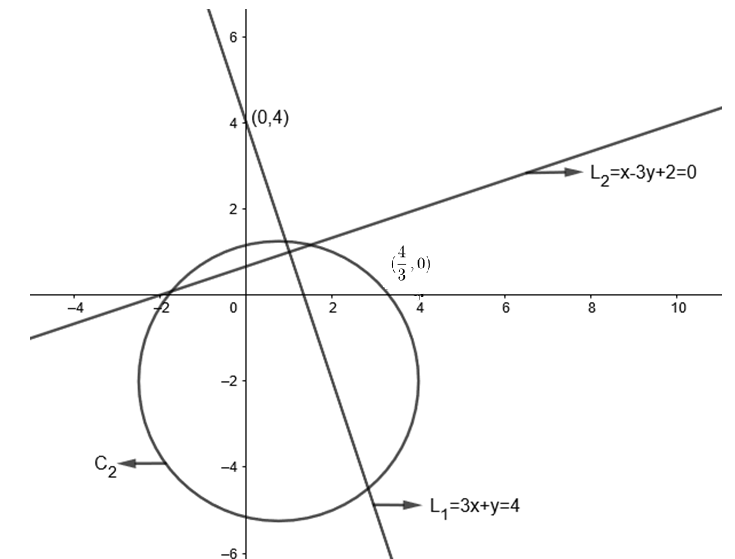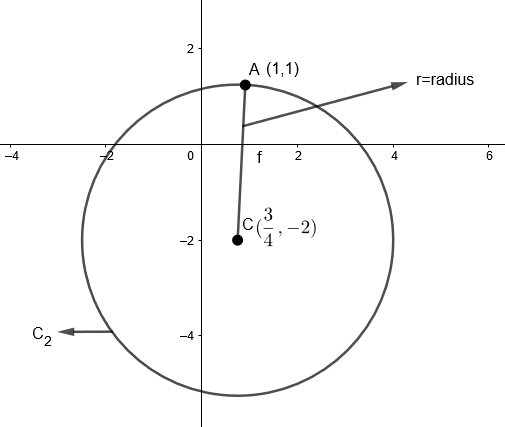Courses
Courses for Kids
Free study material
Free LIVE classes
MoreLIVE
Join Vedantu’s FREE Mastercalss

# Find the equation of the circle passing through the intersection of the lines $3x+y=4$ and $x-3y+2=0$ and concentric with the circle $2\left( {{x}^{2}}+{{y}^{2}} \right)-3x+8y-1=0$.Verified
363.6k+ views
Hint: Two concentric circles have same center and distance from center to any point on circumference is radiusGiven lines, ${{L}_{1}}=3x+y=4....\left( i \right)$
${{L}_{2}}=x-3y+2=0....\left( ii \right)$
We have to find the point of intersection of ${{ L }_{1}}$and ${{L}_{2}}$, we will solve equation $\left( i \right)$and $\left( ii \right)$together.
Taking equation$\left( i \right)$, $3x+y=4$
We get, $y=4-3x....\left( iii \right)$
Putting value of $y$ in equation $\left( ii \right)$
$x-3y+2=0$
$x-3\left[ 4-3x \right]+2=0$
$x-12+9x+2=0$
$10x=10$
Therefore, we get
Putting value of $x$ in equation $\left( iii \right)$to find the value of $y$
$y=4-3x$
$=4-3\left( 1 \right)$
We get $y=1$
Therefore circle ${{C}_{2}}$passes through$\left( x,y \right)=\left( 1,1 \right)$.
Now circle ${{C}_{2}}$is concentric with circle:
${{C}_{1}}=2\left( {{x}^{2}}+{{y}^{2}} \right)-3x+8y-1=0$
Dividing the equation by $2$,
We get ${{C}_{1}}=\left( {{x}^{2}}+{{y}^{2}} \right)-\dfrac{3x}{2}+4y-\dfrac{1}{2}=0....\left( iv \right)$
The general equation of circle is,
${{x}^{2}}+{{y}^{2}}+2gx+2fy+c=0$
Now, we will compare general equation of circle with equation$\left( iv \right)$.
We get, $2g=-\dfrac{3}{2}$
$g=\dfrac{-3}{4}$
$2f=4$
$f=2$
We know that center of circle is $\left( -g,-f \right)$.
Therefore, $\text{centre}=\left( \dfrac{3}{4},-2 \right)$
Since, given circle is concentric with circle to be found $\left( {{C}_{2}} \right)$, both would have same center.
Now, the new circle ${{C}_{2}}$has center at $C\left( \dfrac{3}{4},-2 \right)$and it passes through intersection of line $A=\left( 1,1 \right)$.
Distance of point $A\left( 1,1 \right)$to $C\left( \dfrac{3}{4},-2 \right)$is radius.By distance formula,
$r=AC=\sqrt{{{\left( {{x}_{2}}-{{x}_{1}} \right)}^{2}}+{{\left( {{y}_{2}}-{{y}_{1}} \right)}^{2}}}$
$r=AC=\sqrt{{{\left( 1-\dfrac{3}{4} \right)}^{2}}+{{\left( 1+2 \right)}^{2}}}$
$=\sqrt{\dfrac{1}{16}+9}$
We get radius $=\sqrt{\dfrac{145}{16}}=\dfrac{\sqrt{145}}{4}\text{ units}$
Now we know that, equation of circle with center $\left( a,b \right)$and radius $r$
$={{\left( x-a \right)}^{2}}+{{\left( y-b \right)}^{2}}={{r}^{2}}$
Here, we found the center $\left( \dfrac{3}{4},-2 \right)$and radius$=\dfrac{\sqrt{145}}{4}\text{ units}$
We get, equation of circle $={{\left( x-\dfrac{3}{4} \right)}^{2}}+{{\left[ y-\left( -2 \right) \right]}^{2}}=\dfrac{145}{16}$
$=16{{x}^{2}}+16{{y}^{2}}-24x+64y=72$
Therefore, final equation of circle is:
$2\left( {{x}^{2}}+{{y}^{2}} \right)-3x+8y=9$
Note:
Elimination method is also suitable to find the intersection of line. Students can also use the method of family of circles passing through a point and having a center with constraint.

Last updated date: 19th Sep 2023
Total views: 363.6k
Views today: 5.63k